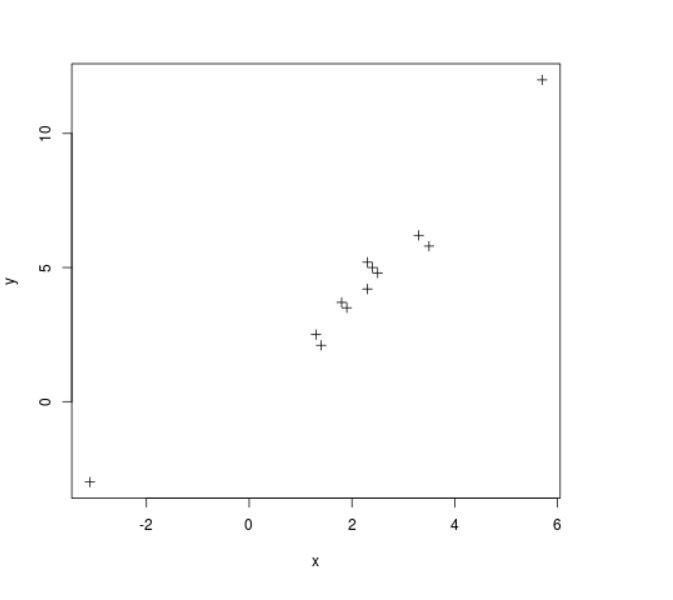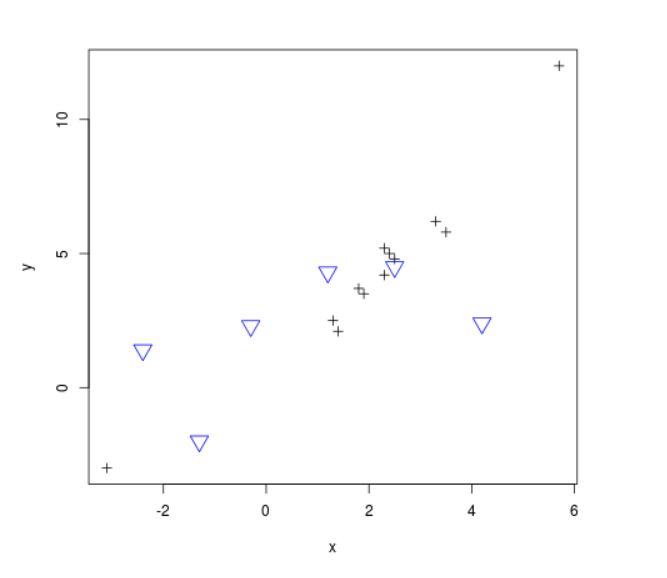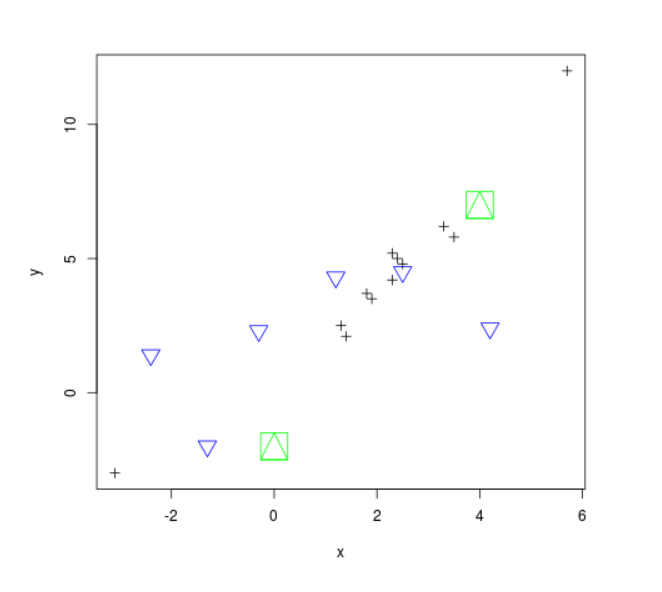# Addition of more points to a Plot in R Programming – points() Function

`points()` function in R Language is used to add a group of points of specified shapes, size and color to an existing plot.

Syntax: points(x, y, cex, pch, col)

Parameters:
x, y: Vector of coordinates
cex: size of points
pch: shape of points
col: color of points

Sample Scatter Plot:

 `# R program to create a scatter plot ` ` `  `# Creating coordinate vectors ` `x <``-` `c(``1.3``, ``3.5``, ``1.4``, ``-``3.1``, ``5.7``, ``2.4``, ``3.3``, ``2.5``, ``2.3``, ``1.9``, ``1.8``, ``2.3``) ` `y <``-` `c(``2.5``, ``5.8``, ``2.1``, ``-``3``, ``12``, ``5``, ``6.2``, ``4.8``, ``4.2``, ``3.5``, ``3.7``, ``5.2``) ` ` `  `# Plotting the graph ` `plot(x, y, cex ``=` `1``, pch ``=` `3``, xlab ``=``"x"``, ylab ``=``"y"``, col ``=``"black"``) `

Output:Here, a sample scatter plot is created for the addition of points.

Example 1: Adding points to scatterplots

 `# R program to add points to a plot ` ` `  `# Creating coordinate vectors ` `x <``-` `c(``1.3``, ``3.5``, ``1.4``, ``-``3.1``, ``5.7``, ``2.4``, ``3.3``, ``2.5``, ``2.3``, ``1.9``, ``1.8``, ``2.3``) ` `y <``-` `c(``2.5``, ``5.8``, ``2.1``, ``-``3``, ``12``, ``5``, ``6.2``, ``4.8``, ``4.2``, ``3.5``, ``3.7``, ``5.2``) ` `plot(x, y, cex ``=` `1``, pch ``=` `3``, xlab ``=``"x"``, ylab ``=``"y"``, col ``=``"black"``) ` ` `  `# Creating coordinates to be added   ` `x2 <``-` `c(``4.2``, ``1.2``, ``-``2.4``, ``-``0.3``, ``-``1.3``, ``2.5``) ` `y2 <``-` `c(``2.4``, ``4.3``, ``1.4``, ``2.3``, ``-``2``, ``4.5``) ` ` `  `# Calling points() function ` `points(x2, y2, cex ``=` `2``, pch ``=` `6``, col ``=``"blue"``) `

Output:Example 2: Adding points of different properties

 `# R program to add points to a plot ` ` `  `# Creating coordinate vectors ` `x <``-` `c(``1.3``, ``3.5``, ``1.4``, ``-``3.1``, ``5.7``, ``2.4``, ``3.3``, ``2.5``, ``2.3``, ``1.9``, ``1.8``, ``2.3``) ` `y <``-` `c(``2.5``, ``5.8``, ``2.1``, ``-``3``, ``12``, ``5``, ``6.2``, ``4.8``, ``4.2``, ``3.5``, ``3.7``, ``5.2``) ` `plot(x, y, cex ``=` `1``, pch ``=` `3``, xlab ``=``"x"``, ylab ``=``"y"``, col ``=``"black"``) ` ` `  `# Creating coordinates to be added   ` `x2 <``-` `c(``4.2``, ``1.2``, ``-``2.4``, ``-``0.3``, ``-``1.3``, ``2.5``) ` `y2 <``-` `c(``2.4``, ``4.3``, ``1.4``, ``2.3``, ``-``2``, ``4.5``) ` ` `  `# Calling points() function ` `points(x2, y2, cex ``=` `2``, pch ``=` `6``, col ``=``"blue"``) ` ` `  `# Adding points of different shape and size ` `x3 <``-` `c(``4``, ``0``) ` `y3 <``-` `c(``7``, ``-``2``) ` ` `  `points(x3, y3, cex ``=` `4``, pch ``=` `14``, col ``=``"green"``) `

Output:My Personal Notes arrow_drop_upCheck out this Author's contributed articles.

If you like GeeksforGeeks and would like to contribute, you can also write an article using contribute.geeksforgeeks.org or mail your article to contribute@geeksforgeeks.org. See your article appearing on the GeeksforGeeks main page and help other Geeks.

Please Improve this article if you find anything incorrect by clicking on the "Improve Article" button below.

Article Tags :

Be the First to upvote.

Please write to us at contribute@geeksforgeeks.org to report any issue with the above content.